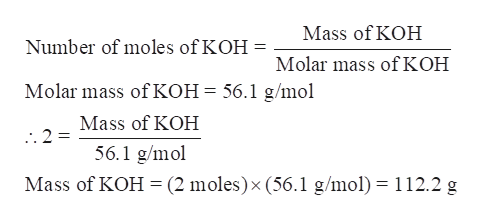# Consider K2O+H2O->2 KOH; how many grams of KOH are produced from 1 mole of K2O?* -> is supposed to be an arrow.

Question
285 views

Consider K2O+H2O->2 KOH; how many grams of KOH are produced from 1 mole of K2O?

* -> is supposed to be an arrow.

check_circle

Step 1

Step 1-

This question is a stoichiometric question. Stoichiometry is based on the law of conservation of mass which states that the total mass of the reactant is equal to the total mass of products i.e. there is no net loss of mass during a chemical reaction.

Step 2

Step 2-

We are given a chemical equation for the reaction of K2O with H2O.

As per the balanced chemical equation, 1 mole of K2O reacts with 1 mole of H2O to produce 2 moles of KOH.

Therefore, moles of KOH produced by 1 mole of K2O are 2.

Step 3

Step 3- from the number of moles of KOH produced; d...help_outlineImage TranscriptioncloseMass of KOH Number of moles of KOH = Molar mass of KOH Molar mass of KOH = 56.1 g/mol Mass of KOH 56.1 g/mol Mass of KOH (2 moles) x (56.1 g/mo) 112.2 g fullscreen

### Want to see the full answer?

See Solution

#### Want to see this answer and more?

Solutions are written by subject experts who are available 24/7. Questions are typically answered within 1 hour.*

See Solution
*Response times may vary by subject and question.
Tagged in

### Chemistry